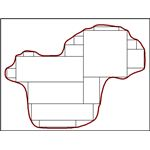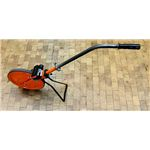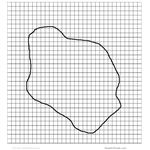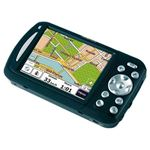Methods of Calculating or Measuring the Area of Land

Surveying irregular and large land tracts can be done by various methods. While some methods are simple and based on basic geometry, others are complex and may involve GPS and satellite imagery. The different options and technologies available to the surveyor have been investigated in this post. Land needs to be measured for various reasons like prior to purchase, when doing stock taking, building a boundary wall, dispute with a neighbor over demarcation, etc. Measuring regular land area like residential plots sold by real estate agents and land developers is easy as they have mostly rectangle or other geometrical shapes. Recalling geometry studied in High School we can do the measurements and arrive at the results. But if the area of land is highly irregularly shaped or if you are purchasing large tracts of land like a farm measuring miles across. Then how do you do the measurements?

Always make use of the services of a land surveyor or a civil engineer? But that is expensive. From time to time you want to measure the area of your land and calculate how much it is worth. My article explains some Â methods of measuring your land and some high technology solutions.

Geometric Method

In geometric method the odd shaped plot is surveyed and plotted over graph paper. Area of the plot is then subdivided into geometric figures whose area we can calculate by formula.

First the largest size geometric figure that can be neatly be drawn in the map is made, followed by smaller shapes, until the complete space has been occupied more or less.

All these figures have their areas calculated, which are then added together to give the total area.

For example:

Area of Rectangle: Length x Breadth

Area of Square: Length x Length

Area of Triangle: 0.5 x Base x Height

Area of Trapezium: 0.5 x (Length 1 + Length 2) x Height

Using these formulas the correct area can be arrived.

Dividing into Geometric ShapesÂ

Using Planimeter on Map

A small device called planimeter is used for measuring areas of a graphically represented planar region. This equipment can measure all regular and irregular shapes. All you have to do is to pivot it at any point and then run the end pin all over the perimeter of the area you want to measure. It has got a flexible linkage which allows it to move without any effort in all directions.

A planimeter is only useful if you have a map of the area or an aerial photograph. Nowadays you can get high resolution photos by satellites by using Google earth, wikimapia, and other applications. You can also draw your own map after you have taken the dimensions and angles of the various lines and afterwards measure it using a planimeter.

One problem that can occur is that of scale. Planimeter will give the area measurement in its own scale or square meter and you have to convert it to your own unit. Having a known area at hand on the map or by drawing a small square within the map you can calculate its area as you know the scale ratio of the map. Then you run the planimeter over it and get its evaluation.

For example if your square is 300 square feet and the planimeter measures it as 1.5 square meter then

1 m2= 200 feet square

Thus multiplying the final reading of the planimeter in m2Â by 2 will give you the reading in square feet.

PlanimeterBy Using Measuring Wheel

A measuring wheel is another method of measuring the area. In principle it is like a planimeter that you have to go over the perimeter of the area with it. Only you have to do it on the actual land. Built like a single bicycle wheel, it has to be run all over the edges of the land to be measured. Gives the reading in units chosen when you reach the starting point.By using Graph Paper

With graph paper the map is superimposed on a translucent sheet or you can take a Photostat on a transparent slide. Then it can be put over a suitable sized graph paper and clamped together. As the area of each square of the graph is known. Measuring the approximate number of squares and multiplying it by the area and the scale will give you the area.

Land Marked on Graph Paper for CalculationUsing GPS

GPS stands for global positioning system and consists of sets of satellites that give your coordinates when you use a hand held receiver. It gives your position coordinates with an accuracy of within 1 meter. Can be used in large land areas where you can go to each end and note the coordinates and later calculate the area.Using Aerial Photographs

The aerial photographs of the land areas can also be used for the measurement by using a planimeter or by graph paper using suitable scale. These are essential in land surveys. This is very useful if the land has visible markings or can be seen properly or having contrast.

Subscribe to OpenW3.com

1.Vijay Kumar says:

Nice elaboration of methods of measurement of areas on drawings of area surveyed.

But it still requires to give reference of any authority or National or international standard which shows that area under river or water body enclosed by land surveyed is not to be deducted form the total area surveyed for the purpose of payment of the total area surveyed.

•Lenny Hudson says:

Thanks Mr. Vijaya Kumar. Point noted and will correct soon

2.LastTerrell says:

I see you don’t monetize your website, don’t waste
your traffic, you can earn additional cash every month because you’ve got
high quality content. If you want to know how to make extra money, search for: Mertiso’s
3.Samuel says: